# Data sufficiency Quiz for RRB PO & RRB CLERK : Quiz – 8

## Data sufficiency Quiz for RRB PO & RRB CLERK : Quiz – 8

In logical reasoning, Data sufficiency questions contain a few statements, and students must choose which of the provided statements will aid in obtaining an answer or solution to the question.

These Data sufficiency questions are typically used to assess a candidate’s logical and analytic abilities. In the reasoning ability, students are usually offered 4-5 data sufficiency problems, which they can readily answer.

This page contains 10 data sufficiency problems from the Reasoning Ability section.
Candidates can practice Data sufficiency topic with help of our Data sufficiency quiz for forthcoming competitive examinations like IBPS clerk, IBPS PO, IBPS RRB PO, IBPS RRB clerk, SBI PO, SBI clerk etc.

Directions (1-3): Each question below is followed by two statements I and II. You have to determine whether the data given in the statement is sufficient to answer the question.
1. If “Kamal and Raghav are friends” is coded as “mr ea sf ua ad”, in a certain code language. Then how “hockey” is coded in that language?
I. “Kamal plays cricket” is coded as “kr mr ps”.
II. “Raghav plays hockey” is coded as “ua ce ps”.
(a) If the statement I alone is sufficient to answer the question, but the statement II alone is not sufficient.
(b) If the statement II alone is sufficient to answer the question, but the statement I alone is not sufficient.
(c) If either the statement I alone or the statement II alone is sufficient to answer the question.
(d) If neither of the statements are sufficient to answer the question.
(e) If both statements I and II together are needed to answer the question.2. Who is the tallest among A, B, C, D and E.
I. C is shorter than D but taller than at least two people, E is shorter than B.
II. D is taller than at least 3 people. A is taller than E and C.
(a) If the statement I alone is sufficient to answer the question, but the statement II alone is not sufficient.
(b) If the statement II alone is sufficient to answer the question, but the statement I alone is not sufficient.
(c) If either the statement I alone or the statement II alone is sufficient to answer the question.
(d) If neither of the statements are sufficient to answer the question.
(e) If both statements I and II together are needed to answer the question.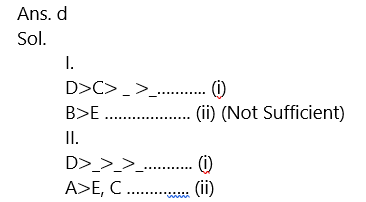Directions (4-6): Study the information carefully and answer the questions given below.
4. Point X is in which direction with respect to J?
I. Point J is to the east of point V, which is the south of point T. Point X is to the south of point W.
II. Point T is to the west of point Y, which is to the north of point W.
(a) The data in both statements I and II together are necessary to answer the question.
(b) The data in statement II alone are sufficient to answer the question while the data in statement
I alone are not sufficient to answer the question.
(c) The data even in both statements I and II together are not sufficient to answer the question.
(d) The data either in statement I alone or in statement II alone are sufficient to answer the question.
(e) The data in statement I alone are sufficient to answer the question, while the data in statement II alone are not sufficient to answer the question.

Ans. c
Sol. Even both the statement together is not sufficient to answer the question.

5. Eight students A, B, C, D, E, F, G and H are sitting around a circle facing to the centre of the table. How many students are sitting between C and F?
I. A is seated second to the left of H, who is seated second to the left of D. G is seated second to the right of F.
II. C is seated third to the right of A and is not near to E. F is seated second to the right B. D is seated second to the left of E and is third to right of G. C is an immediate neighbor of H.
(a) The data in both statements I and II together are necessary to answer the question.
(b) The data in statement II alone are sufficient to answer the question while the data in statement
I alone are not sufficient to answer the question.
(c) The data even in both statements I and II together are not sufficient to answer the question.
(d) The data either in statement I alone or in statement II alone are sufficient to answer the question.
(e) The data in statement I alone are sufficient to answer the question, while the data in statement II alone are not sufficient to answer the question.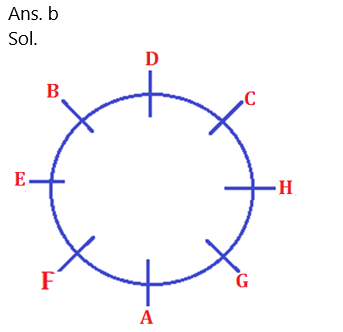6. A dealer sold six watches A, B, C, D, E and F during a period of Monday to Saturday, one watch on each day. The watch A was sold immediately after?
I. The watch C was sold at least before three watches. The watch F was sold on Tuesday. The watch D was sold immediately after the watch C.
II. At least four watches were sold after the watch E. Both the watches B and E were sold at least before one watch.
(a) The data in both statements I and II together are necessary to answer the question.
(b) The data in statement II alone are sufficient to answer the question while the data in statement I alone are not sufficient to answer the question.
(c) The data even in both statements I and II together are not sufficient to answer the question.
(d) The data either in statement I alone or in statement II alone are sufficient to answer the question.
(e) The data in statement I alone are sufficient to answer the question, while the data in statement II alone are not sufficient to answer the question.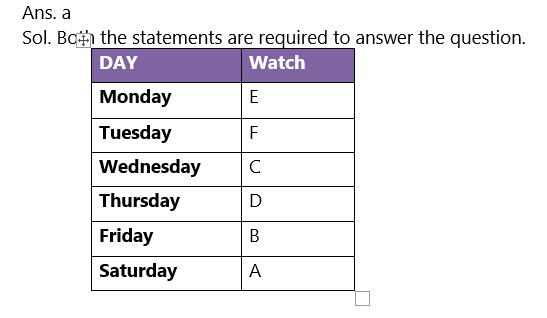Directions (7-10): Each of the questions below consists of a question and two statements numbered I and II given below it. You have to decide whether the data provided in the statement are sufficient to answer the question. Read both the statements and Give answer:

7. On which of the following day of the week starting from Monday to Sunday Riya leaves for Puducherry?
I. Riya’s sister correctly remembers that Riya left for Puducherry one of the day after Wednesday.
II. Riya’s mother correctly remembers that Riya left for Puducherry after Tuesday but before Saturday.
(a) If the data in statement I alone are sufficient to answer the question, while the data in statement II alone are not sufficient to answer the question.
(b) If the data in statement II alone are sufficient to answer the question, while the data in statement I alone are not sufficient to answer the question.
(c) If the data either in statement I alone or in statement II alone are sufficient to answer the question.
(d) If the data even in both statements I and II together are not sufficient to answer the question.
(e) If the data in both statements I and II together are necessary to answer the question.

Ans. d
Sol. From statement I, Riya leave for Puducherry after Wednesday which may be either on Thursday, Friday, Saturday or on Sunday.
From statement II, Riya leave for Puducherry either on Wednesday, Thursday or on Friday.
And even after combining these two statements we don’t get the confirm day on which Riya leave for Puducherry as it may be on Thursday or on Friday.

8. Who among the following Ashu, Ravi, Neha, Nitin and Riya got the highest marks in the examination (out of 100)?
I. The one who got 75 marks is the person who got second highest marks in the examination. Ashu scored more marks than Neha and Riya. Ashu does not got the highest marks.
II. Riya get more marks than only one person. Neha got more marks than Riya but not the second highest marks.
(a) If the data in statement I alone are sufficient to answer the question, while the data in statement II alone are not sufficient to answer the question.
(b) If the data in statement II alone are sufficient to answer the question, while the data in statement I alone are not sufficient to answer the question.
(c) If the data either in statement I alone or in statement II alone are sufficient to answer the question.
(d) If the data even in both statements I and II together are not sufficient to answer the question.
(e) If the data in both statements I and II together are necessary to answer the question.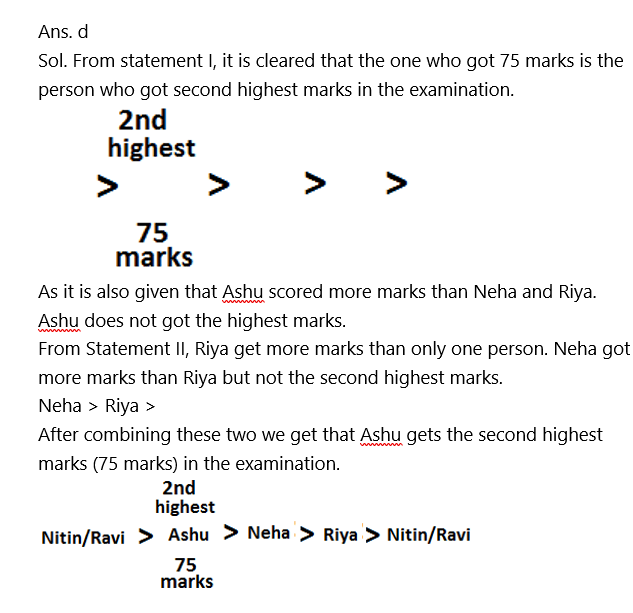9. Some persons are sitting in a row facing in the same direction. How many persons are sitting in the row?
I. A sits third to the left of G, and second to the right of M. Both M and G does not sit at the end of the row. B sits at the end of the row.
II. L sits fourth to the left of E and sits at the end of the row. B sits fourth to the right of K, who sits second to the right of A. E, sits between M and A.
(a) If the data in statement I alone are sufficient to answer the question, while the data in statement II alone are not sufficient to answer the question.
(b) If the data in statement II alone are sufficient to answer the question, while the data in statement I alone are not sufficient to answer the question.
(c) If the data either in statement I alone or in statement II alone are sufficient to answer the question.
(d) If the data even in both statements I and II together are not sufficient to answer the question.
(e) If the data in both statements I and II together are necessary to answer the question.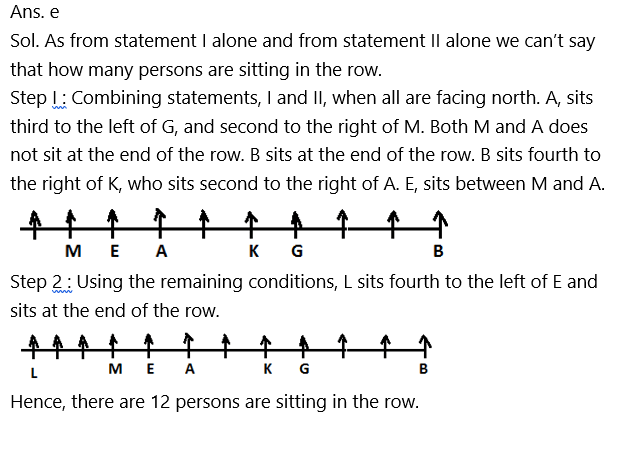10. What is the rank of Adarsh from the left in the class of 45 students?
I. Rahul’s rank is 16th from the left. Adarsh sits in between Rahul and Aman. Aman’s rank is 15th from the right.
II. Rahul’s rank from the right is 19th from the right. Adarsh sits on the left of Rahul. There are seven persons sits between Ritu and Rahul. Adarsh sits exactly between Ritu and Rahul.
(a) If the data in statement I alone are sufficient to answer the question, while the data in statement II alone are not sufficient to answer the question.
(b) If the data in statement II alone are sufficient to answer the question, while the data in statement I alone are not sufficient to answer the question.
(c) If the data either in statement I alone or in statement II alone are sufficient to answer the question.
(d) If the data even in both statements I and II together are not sufficient to answer the question.
(e) If the data in both statements I and II together are necessary to answer the question.

Ans. b
Sol. From Statement I, Rahul’s rank is 16th from the left. Adarsh sits exactly between Rahul and Aman. Aman’s rank is 15th from the right. So, Aman’s rank is (45-15+1)= 31st from the left. There are total fourteen students between Rahul and Aman and Adarsh sits between Rahul and Aman. Hence, Adarsh rank is either 23rd or 24th from the left.

From statement II, Rahul’s rank from the right is 19th from the right and (45-19+1)= 27th from the left. Adarsh sits on the left of Rahul. There are seven persons sits between Ritu and Rahul. Adarsh sits exactly between Ritu and Rahul. So Ritu is on the left of Rahul. Ritu’s rank from the left is (rank of Rahul-7-1)= (27-8)=19th from the left. And as there are seven persons between Ritu and Rahul and Adarsh is exactly between Ritu and Rahul, So Adarsh is either 4th on the left of Rahul or 4th on the right of Ritu.
Hence, Adarsh rank from the left is (19+4)= 23rd from the left.

#### Attempt Quantitative Aptitude Topic Wise Online Test Series

Recommended PDF’s for:

#### Most important PDF’s for Bank, SSC, Railway and Other Government Exam : Download PDF Now

AATMA-NIRBHAR Series- Static GK/Awareness Practice Ebook PDF Get PDF here
The Banking Awareness 500 MCQs E-book| Bilingual (Hindi + English) Get PDF here
AATMA-NIRBHAR Series- Banking Awareness Practice Ebook PDF Get PDF here
Computer Awareness Capsule 2.O Get PDF here
AATMA-NIRBHAR Series Quantitative Aptitude Topic-Wise PDF Get PDF here
AATMA-NIRBHAR Series Reasoning Topic-Wise PDF Get PDF Here
Memory Based Puzzle E-book | 2016-19 Exams Covered Get PDF here
Caselet Data Interpretation 200 Questions Get PDF here
Puzzle & Seating Arrangement E-Book for BANK PO MAINS (Vol-1) Get PDF here
ARITHMETIC DATA INTERPRETATION 2.O E-book Get PDF here
3